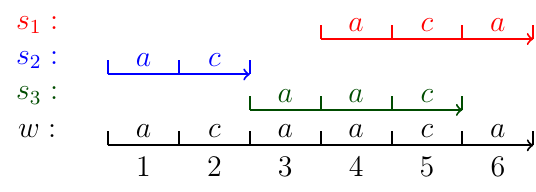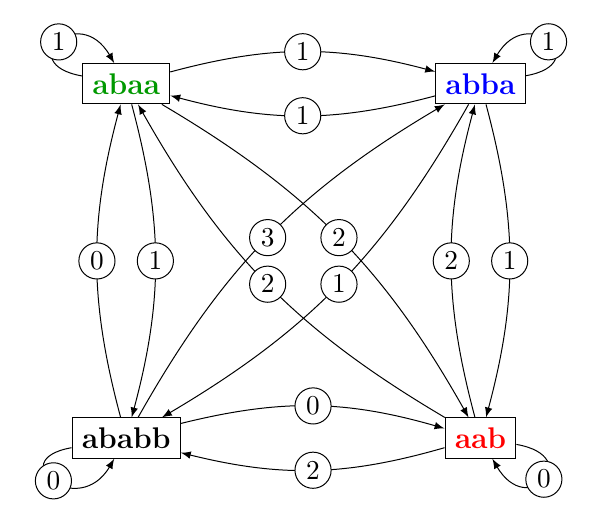# Superstring

 Author B. Cazaux & E. Rivals (rivals@lirmm.fr) Date 2018-04-10

Abstract: This document covers some knowledge and research results about the algorithmic notion of superstring.

## 1 Introduction to superstring

Strings (also called words), which are sequence of symbols, arise in many applications. Superstrings result from merging strings that overlap.Figure 1: Superstring of three words

In this example, $$w$$ is a superstring of the three words $$s_1$$, $$s_2$$, and $$s_3$$. As seen in the above example: $$s_3$$ overlaps $$s_1$$ by 2 symbols. This overlap is "used" to merge $$s_1$$, and $$s_3$$.

### 1.1 The archetypical question: Shortest Linear Superstring.

A well-studied question in algorithms (in computer science) is called Shortest Linear Superstring.

You are given a set of words, say $$P$$. We denote the words of $$P$$ by $$s_1, \ldots, s_n$$. In other words, the input is $$P := \{s_1, \ldots, s_n\}$$ a set of words. The sum of their length is denoted by $$\vert \vert P \vert \vert := \sum_1^n\left| s_i \right|$$ and called the norm of $$P$$.

The question is: find a string $$w$$ that is a superstring of all words of $$P$$ and is a short as possible.

In a sense, this question is difficult to solve.

### 1.2 The Overlap Graph (OG)

One way to solve it is to build a directed graph called the Overlap Graph for $$P$$. In this graph, each input word is represented by a node. An arc from a node $$x$$ to a node $$y$$ is weighted by the length of the longest overlap from word $$x$$ onto word $$y$$. Once the OG is built, it suffices to search a special kind of path in this graph. The desired type of path is known as a Maximum Weighted Hamiltonian Path. It must visit all nodes once and only once, and moreover, the sum of the weights of the arcs it traverses must be maximal.Figure 2: Example of overlap graph for $$P := \{abaa, abba, ababb, aab\}$$

Game

1. Can you find a Hamiltonian path on this graph?
2. Can you find a Hamiltonian path on this graph, such that the sum of the weigths on its arcs is the largest?

## 2 The Hierarchical Overlap Graph

We have proposed another graph that can replace the Overlap Graph, it is called the Hierarchical Overlap Graph (HOG).

An algorithm to build the HOG for a set of words is presented in this preprint:

B. Cazaux, E. Rivals. Hierarchical Overlap Graph. HAL Archives ouvertes <lirmm-01674319> 2017.

An appendix to this manuscript is available here: http://www.lirmm.fr/~rivals/res/superstring/hog-art-appendix.pdf

## 3 Practical bounds of an optimal shortest linear superstrings

As computing an optimal superstring for the Shortest Linear Superstring question is difficult, researchers have proposed approximation algorithms. These compute a superstring that is not guaranteed to be optimal, but is close to an optimal superstring. Fews of these approximation algorithms have been implemented and tested in practice. Hence, it remains to determine which algorithms work well in practice and on which types of instances.

In 2017, we proposed an algorithm (and a program implementing it) that computes a lower and an upper bound on the length of a shortest linear superstring of an input set $$P$$. The implementation of this algorithm called MGreedyMin is a result from the Master thesis of Samuel Juhel (2017). Both the algorithm and details of its implementation are presented in:

B. Cazaux, S. Juhel, E. Rivals. Practical bounds of an optimal shortest linear superstrings. In proceedings of 17th International Symposium on Experimental Algorithms (SEA 2018)}. LIPICs series, vol. 103, article 18, 2018.

An appendix to this manuscript is available here: http://www.lirmm.fr/~rivals/res/superstring/sls-practical-bounds-appendix.pdf

In practice, two main results emerge from this study:

1. the program that implements MGreedyMin scales up well and can process very large input sets containing millions of words whose total length sums up to hundreds of millions of symbols.
2. the computed lower and upper bounds are extremely close in practice compared to the size of the input.

## 4 Collaborators and contributors.

The work and studies presented and mentioned in this page have been achieved with help of colleagues with who we collaborated. We wish to thank them here.

• Samuel Juhel, was a master student at Montpellier University in 2017. He works on practical bounds for SLS and collaborated to the article published in SEA 2018.
• Rodrigo Cànovas, postdoctoral fellow at IBC (2015-2017), has co-written the work on the first data structure that implements the Extended Hierarchical Overlap Graph (which serves as an intermediate graph to build the HOG). He has implemented it in an index termed COvI. COvI is used to compute the EHOG in the article published at SEA 2018.

## 5 Abbreviations

• SLS: Shortest Linear Superstring
• HOG: Hierarchical Overlap Graph
• EHOG: Extended Hierarchical Overlap Graph
• COvI: Compressed Overlap Index
• IBC: Institute of Computational Biology

Created: 2018-04-10 mar. 12:37

Emacs 25.2.2 (Org mode 8.3.6)

Validate